## Friday, 8 August 2014

### CHAPTER 3-Subtraction of Vectors

Consider two vectors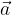$\vec a$ and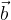$\vec b$; we wish to find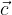$\vec c$ such that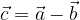$\vec c = \vec a - \vec b$
We can slightly modify this relation and write it as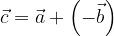$\vec c = \vec a + \left( { - \vec b} \right)$
and thus subtraction can be treated as addition. To do this, we first reverse the vector$\vec b$ to obtain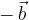$- \,\vec b$ and then use the triangle / parallelogram law of addition to add the vector$\vec a$ and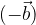$( - \vec b)$:Joining the tip of$\vec b$ to the tip of$\vec a$ (if$\vec a$ and$\vec b$ are co-initial) also gives us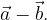$\vec a - \vec b.$
Note that from the triangle law, it follows that for three vectors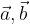$\vec a,\vec b$ and$\vec c$representing the sides of a triangle as shown,We must have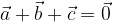$\vec a + \vec b + \vec c = \vec 0$
In fact, for the vectors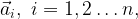${\vec a_i},\,\,i = 1,2\ldots n,$ representing the sides of an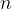$n$-sided polygon as shown,we must have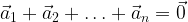${\vec a_1} + {\vec a_2} + \ldots+ {\vec a_n} = \vec 0$
since the net effect of all vectors is to bring us back from where we started, and thus our net displacement is the zero vector.